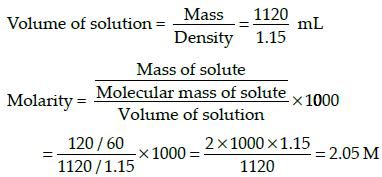# Test: Past Year Questions Of Basic Concept Of Chemistry

## 20 Questions MCQ Test Chemistry for JEE | Test: Past Year Questions Of Basic Concept Of Chemistry

Description
This mock test of Test: Past Year Questions Of Basic Concept Of Chemistry for JEE helps you for every JEE entrance exam. This contains 20 Multiple Choice Questions for JEE Test: Past Year Questions Of Basic Concept Of Chemistry (mcq) to study with solutions a complete question bank. The solved questions answers in this Test: Past Year Questions Of Basic Concept Of Chemistry quiz give you a good mix of easy questions and tough questions. JEE students definitely take this Test: Past Year Questions Of Basic Concept Of Chemistry exercise for a better result in the exam. You can find other Test: Past Year Questions Of Basic Concept Of Chemistry extra questions, long questions & short questions for JEE on EduRev as well by searching above.
QUESTION: 1

### 20 ml of decinormal solution of NaOH neutralises 25 ml of a solution of dibasic acid containing 3g. of the acid per 500 ml. The Molecular weight of the acid is

Solution:

NaOH = dibasic acid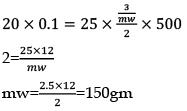QUESTION: 2

### In a compound C, H and N are present in the ratio 9: 1: 3.5 by weight. Molecular mass of the compound is 108. Molecular formula of the compound is:

Solution:

C% = 9/12 = 0.75 = 0.75/0.25 = 3
H% 1/1 =1 = 1/0.25 = 4

N= 3.5/14 = 0.25 = 0.25/0.25 = 1
Empirical formula = C3H4N
36+4+14=54
n = 108/54 = 2
(C3H4N) × 2
= C6H8N2

QUESTION: 3

### Number of atoms is 560 g of Fe (atomic mass = 56 g mol-1)

Solution:

Fe atom= 560/56 =10 mole
= 10 x NA atom
option(A) = 5 mol
5 x NA
option (B) = 20/1 = 20
20 x NA

QUESTION: 4

What volume of hydrogen gas at 273 K and 1 atm pressure will be consumed in obtaining 21.6 g elemental boron (atomic mass = 10.8) from the reduction of  boron trichloride by hydrogen?

Solution:

BCl3 + 3H ⇋ B+3HCl
BCl3 + 3/2 → B + 3HCl
3/2 mol → 1 mol
1 mol B → 3/2 molH2
2 mole → 3/2  x 2 = 6/2 = 3 mole
1 mole = 22.4 liter S.T.P
3----?
= 22.4 x 3
=67.2 litre

QUESTION: 5

One mole of magnesium nitride on reaction with an excess of water gives

Solution:

Mg3H2 + 3H2O→ 3Mg(OH) 2 + 2NH3

QUESTION: 6

How many moles of magnesium phosphate, Mg3 (PO4)2 will contain 0.25 mole of oxygen atoms?

Solution:

Mg3(P04)→ 3Mg
→2 P
→ 8 mole oxygen
8 mole of oxygen - 1 mole Mg3 (P0)2
0.25 - ? 0.25/8 = 3 x 10-2

QUESTION: 7

In the reaction, 2Al (s) + 6HCl (aq) → 2Al3+ (aq) + 6Cl- (aq) + 3H2(g)

Solution:

2AI + 6HCl  →  2A l3+ + 6Cl- + 3H2
6 ----- 3 mol
1 mol ---- ?
3/6 = 1/2
= 0.5
At S.T.P= 22.4 - 1 mol
11.2 L

QUESTION: 8

Volume occupied by one molecule of water (density = 1 g cm-3) is

Solution:

Answer:

Given

Density of water (ρ) = 1g/cm3

Molar mass of water (M) = 18 g/mol

∴ Molar volume (Vm) = M/ρ

Vm = 18 cm3/mol

∴ Volume of 1 molecule of water = Vm/NA

Where NA is Avogadro number

Volume of 1 molecule of water = 18/(6.022 × 1023)

Volume of 1 molecule of water = 2.989 × 10-23 ≈ 3 × 10-23.

QUESTION: 9

How many moles of lead (II) chloride will be formed from a reaction between 6.5 g of Pbo and 3.2 g of HCl?

Solution:

PbO + 2HCl
6.5\223
= 2.9 x 10-2
= 2.9x10-2 - x
nx = 9a
PbCl2 + H2O
3.2/36.5
= 8.76 x 10-2
8.76 x 10-2 - x
x = 2.9 x 10-2

QUESTION: 10

100 ml of PH3 on heating forms P and H2. The volume change in the reaction is

Solution:

PH⇋ P5 + 3/2 H2
1 mol 1+ 3/2 =2.5 mol
⟹ 100ml - 3/2 = 150ml
⟹150 - 100 = 50ml

QUESTION: 11

Excess of carbon dioxide is passed through 50 mL of 0.5 M calcium hydroxide solution. After the composition of the reaction, the solution was evaporated to dryness. The solid calcium carbonate was completely neutralized with 0.1 N hydrochloric acid. The volume of the hydrochloric acid required is___________

Solution:

N1V1   =  N2V2
50 x 01 = 0.1 x Vml
Vm = 500ml

QUESTION: 12

25.3 g of sodium carbonate, Na2CO3 is dissolved in enough water to make 250 mL of solution. If sodium carbonate dissociates completely, molar concentration of sodium ions, Na+ and carbonate ions,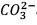are respectively. (At. mass of carbon = 40)

Solution: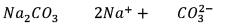= 2.53/106   2.59 × 10-2  5.9 × 10-2
0.238 × 0.25          = 1.18 × 10-3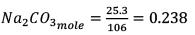250 ml - 0.238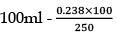= 0.955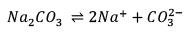0.955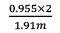0.955

QUESTION: 13

The number of atoms in 0.1 mol of a triatomic gas is

Solution:

Number of atoms = number of moles x Nx atomicity

= 0.1 x 6.02 x 1023 x 3

= 1.806 x 1023

QUESTION: 14

How much time (in hours) would it take to distribute one Avogadro number of wheat grains if 1020 grains are distributed each second?

Solution:

6 x 1023 gram wheat

1 sec → 102O gm distributed

1 hour - 3600sec - 1020 x 3600

= 3.6 x 1023 wheat

3.6 x 1023g  — 1 hour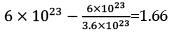QUESTION: 15

For a reaction A + 2B → C, the amount of C formed by starting the reaction with 5 moles of A and 8 moles of B is

Solution:

A +2B → C

t = 0  5    8    0

t= ?  5x  8-2x  xnx = 9a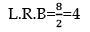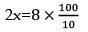x = 4
mole of C = 4 mole

QUESTION: 16

A mixture of CaCl2 and NaCl weighing 4.44 g is treated with sodium carbonate solution to precipitate all the Ca2+ ions as calcium carbonate. The CaCO3 so obtained is heated strongly to get 0.56 g of CaO. The percentage of NaCl in the mixture is

Solution:

CaCl2 + NaCl + NaC0→ CaC03
4.44     3.44
Co.C03milli  = CaCO3milli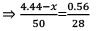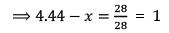x = 3.44
%purity= 3.44/4.44 x 100
=75%

QUESTION: 17

If 1 mL of water contains 20 drops, then number of molecules in a drop of water is

Solution:

1/18 = 5.5 x 10-2(mole of H20
20 drops - 5.5 x 10-2 mole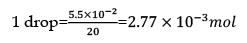= 2.77 x 10-3 x 6.023 x 1023
= 0.0167 x 1023
1.67 x 1021

QUESTION: 18

In an experiment, 4 g of M2Ox oxide was reduced to 2.8g of the metal.Tf the atomic mass of the metal is 56 g mol-1, the number of O atoms in the oxide is

Solution:

M20x → m
W1/E1 = W2/E2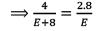⟹ 4E = 2.8E + 22.4
1.2E = 22.4
E= 22.4/1.2 = 18.6
Eq = mw/VF  = 18.6= 56/VF
M203    VF=3
The number of O atoms is 3

QUESTION: 19

Which has the maximum number of molecules among the following?

Solution:

1) 44/44 = 1 mole C02
2) 48/48 =1 mole
3) 8/2 = 4 mole H2
4) 64/64 =1 mole

QUESTION: 20

The density of a solution prepared by dissolving 120 g of urea in 1000 g of water is 1.15 g/ml. The molarity of this solution is:

Solution:

Mass of solute taken = 120g
Molecular mass of solute = 60u
Mass of solvent = 1000g
Density of solution = 1.15g/mL
Total mass of solution = 1000 + 120 = 1120g